# Image classification

This tutorial shows how to classify images of flowers using a `tf.keras.Sequential` model and load data using `tf.keras.utils.image_dataset_from_directory`. It demonstrates the following concepts:

• Identifying overfitting and applying techniques to mitigate it, including data augmentation and dropout.

This tutorial follows a basic machine learning workflow:

1. Examine and understand data
2. Build an input pipeline
3. Build the model
4. Train the model
5. Test the model
6. Improve the model and repeat the process

In addition, the notebook demonstrates how to convert a saved model to a TensorFlow Lite model for on-device machine learning on mobile, embedded, and IoT devices.

## Setup

Import TensorFlow and other necessary libraries:

``````import matplotlib.pyplot as plt
import numpy as np
import PIL
import tensorflow as tf

from tensorflow import keras
from tensorflow.keras import layers
from tensorflow.keras.models import Sequential
``````
```2023-10-27 06:06:38.580862: E external/local_xla/xla/stream_executor/cuda/cuda_dnn.cc:9261] Unable to register cuDNN factory: Attempting to register factory for plugin cuDNN when one has already been registered
2023-10-27 06:06:38.580905: E external/local_xla/xla/stream_executor/cuda/cuda_fft.cc:607] Unable to register cuFFT factory: Attempting to register factory for plugin cuFFT when one has already been registered
2023-10-27 06:06:38.582437: E external/local_xla/xla/stream_executor/cuda/cuda_blas.cc:1515] Unable to register cuBLAS factory: Attempting to register factory for plugin cuBLAS when one has already been registered
```

This tutorial uses a dataset of about 3,700 photos of flowers. The dataset contains five sub-directories, one per class:

``````flower_photo/
daisy/
dandelion/
roses/
sunflowers/
tulips/
``````
``````import pathlib

data_dir = tf.keras.utils.get_file('flower_photos.tar', origin=dataset_url, extract=True)
data_dir = pathlib.Path(data_dir).with_suffix('')
``````
```Downloading data from https://storage.googleapis.com/download.tensorflow.org/example_images/flower_photos.tgz
228813984/228813984 [==============================] - 1s 0us/step
```

After downloading, you should now have a copy of the dataset available. There are 3,670 total images:

``````image_count = len(list(data_dir.glob('*/*.jpg')))
print(image_count)
``````
```3670
```

Here are some roses:

``````roses = list(data_dir.glob('roses/*'))
PIL.Image.open(str(roses))
````````````PIL.Image.open(str(roses))
``````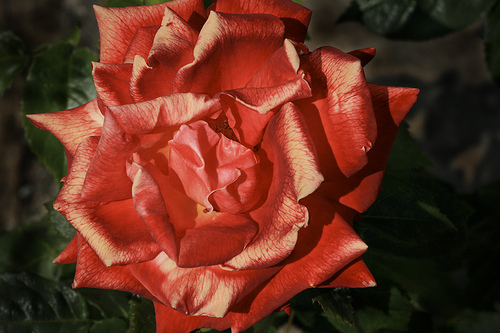And some tulips:

``````tulips = list(data_dir.glob('tulips/*'))
PIL.Image.open(str(tulips))
````````````PIL.Image.open(str(tulips))
``````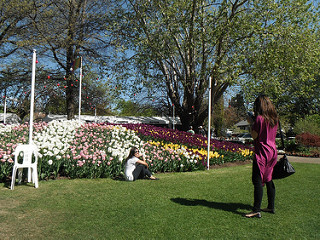## Load data using a Keras utility

Next, load these images off disk using the helpful `tf.keras.utils.image_dataset_from_directory` utility. This will take you from a directory of images on disk to a `tf.data.Dataset` in just a couple lines of code. If you like, you can also write your own data loading code from scratch by visiting the Load and preprocess images tutorial.

### Create a dataset

Define some parameters for the loader:

``````batch_size = 32
img_height = 180
img_width = 180
``````

It's good practice to use a validation split when developing your model. Use 80% of the images for training and 20% for validation.

``````train_ds = tf.keras.utils.image_dataset_from_directory(
data_dir,
validation_split=0.2,
subset="training",
seed=123,
image_size=(img_height, img_width),
batch_size=batch_size)
``````
```Found 3670 files belonging to 5 classes.
Using 2936 files for training.
```
``````val_ds = tf.keras.utils.image_dataset_from_directory(
data_dir,
validation_split=0.2,
subset="validation",
seed=123,
image_size=(img_height, img_width),
batch_size=batch_size)
``````
```Found 3670 files belonging to 5 classes.
Using 734 files for validation.
```

You can find the class names in the `class_names` attribute on these datasets. These correspond to the directory names in alphabetical order.

``````class_names = train_ds.class_names
print(class_names)
``````
```['daisy', 'dandelion', 'roses', 'sunflowers', 'tulips']
```

## Visualize the data

Here are the first nine images from the training dataset:

``````import matplotlib.pyplot as plt

plt.figure(figsize=(10, 10))
for images, labels in train_ds.take(1):
for i in range(9):
ax = plt.subplot(3, 3, i + 1)
plt.imshow(images[i].numpy().astype("uint8"))
plt.title(class_names[labels[i]])
plt.axis("off")
``````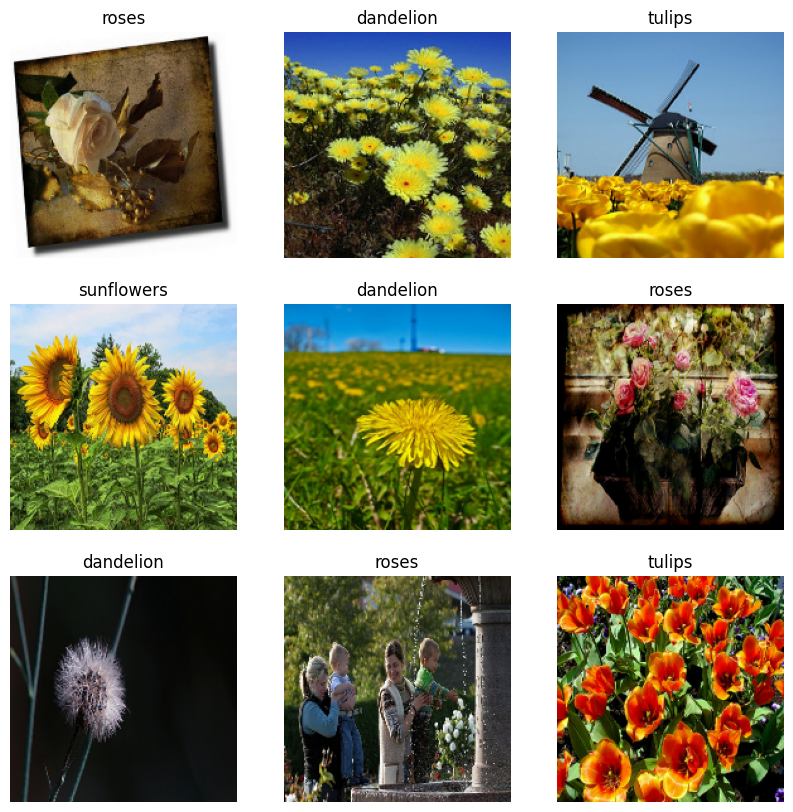You will pass these datasets to the Keras `Model.fit` method for training later in this tutorial. If you like, you can also manually iterate over the dataset and retrieve batches of images:

``````for image_batch, labels_batch in train_ds:
print(image_batch.shape)
print(labels_batch.shape)
break
``````
```(32, 180, 180, 3)
(32,)
```

The `image_batch` is a tensor of the shape `(32, 180, 180, 3)`. This is a batch of 32 images of shape `180x180x3` (the last dimension refers to color channels RGB). The `label_batch` is a tensor of the shape `(32,)`, these are corresponding labels to the 32 images.

You can call `.numpy()` on the `image_batch` and `labels_batch` tensors to convert them to a `numpy.ndarray`.

## Configure the dataset for performance

Make sure to use buffered prefetching, so you can yield data from disk without having I/O become blocking. These are two important methods you should use when loading data:

• `Dataset.cache` keeps the images in memory after they're loaded off disk during the first epoch. This will ensure the dataset does not become a bottleneck while training your model. If your dataset is too large to fit into memory, you can also use this method to create a performant on-disk cache.
• `Dataset.prefetch` overlaps data preprocessing and model execution while training.

Interested readers can learn more about both methods, as well as how to cache data to disk in the Prefetching section of the Better performance with the tf.data API guide.

``````AUTOTUNE = tf.data.AUTOTUNE

train_ds = train_ds.cache().shuffle(1000).prefetch(buffer_size=AUTOTUNE)
val_ds = val_ds.cache().prefetch(buffer_size=AUTOTUNE)
``````

## Standardize the data

The RGB channel values are in the `[0, 255]` range. This is not ideal for a neural network; in general you should seek to make your input values small.

Here, you will standardize values to be in the `[0, 1]` range by using `tf.keras.layers.Rescaling`:

``````normalization_layer = layers.Rescaling(1./255)
``````

There are two ways to use this layer. You can apply it to the dataset by calling `Dataset.map`:

``````normalized_ds = train_ds.map(lambda x, y: (normalization_layer(x), y))
image_batch, labels_batch = next(iter(normalized_ds))
first_image = image_batch
# Notice the pixel values are now in `[0,1]`.
print(np.min(first_image), np.max(first_image))
``````
```0.0 1.0
```

Or, you can include the layer inside your model definition, which can simplify deployment. Use the second approach here.

## A basic Keras model

### Create the model

The Keras Sequential model consists of three convolution blocks (`tf.keras.layers.Conv2D`) with a max pooling layer (`tf.keras.layers.MaxPooling2D`) in each of them. There's a fully-connected layer (`tf.keras.layers.Dense`) with 128 units on top of it that is activated by a ReLU activation function (`'relu'`). This model has not been tuned for high accuracy; the goal of this tutorial is to show a standard approach.

``````num_classes = len(class_names)

model = Sequential([
layers.Rescaling(1./255, input_shape=(img_height, img_width, 3)),
layers.MaxPooling2D(),
layers.MaxPooling2D(),
layers.MaxPooling2D(),
layers.Flatten(),
layers.Dense(128, activation='relu'),
layers.Dense(num_classes)
])
``````

### Compile the model

For this tutorial, choose the `tf.keras.optimizers.Adam` optimizer and `tf.keras.losses.SparseCategoricalCrossentropy` loss function. To view training and validation accuracy for each training epoch, pass the `metrics` argument to `Model.compile`.

``````model.compile(optimizer='adam',
loss=tf.keras.losses.SparseCategoricalCrossentropy(from_logits=True),
metrics=['accuracy'])
``````

### Model summary

View all the layers of the network using the Keras `Model.summary` method:

``````model.summary()
``````
```Model: "sequential"
_________________________________________________________________
Layer (type)                Output Shape              Param #
=================================================================
rescaling_1 (Rescaling)     (None, 180, 180, 3)       0

conv2d (Conv2D)             (None, 180, 180, 16)      448

max_pooling2d (MaxPooling2  (None, 90, 90, 16)        0
D)

conv2d_1 (Conv2D)           (None, 90, 90, 32)        4640

max_pooling2d_1 (MaxPoolin  (None, 45, 45, 32)        0
g2D)

conv2d_2 (Conv2D)           (None, 45, 45, 64)        18496

max_pooling2d_2 (MaxPoolin  (None, 22, 22, 64)        0
g2D)

flatten (Flatten)           (None, 30976)             0

dense (Dense)               (None, 128)               3965056

dense_1 (Dense)             (None, 5)                 645

=================================================================
Total params: 3989285 (15.22 MB)
Trainable params: 3989285 (15.22 MB)
Non-trainable params: 0 (0.00 Byte)
_________________________________________________________________
```

### Train the model

Train the model for 10 epochs with the Keras `Model.fit` method:

``````epochs=10
history = model.fit(
train_ds,
validation_data=val_ds,
epochs=epochs
)
``````
```Epoch 1/10
WARNING: All log messages before absl::InitializeLog() is called are written to STDERR
I0000 00:00:1698386810.987968  496306 device_compiler.h:186] Compiled cluster using XLA!  This line is logged at most once for the lifetime of the process.
92/92 [==============================] - 6s 33ms/step - loss: 1.2684 - accuracy: 0.4554 - val_loss: 1.1000 - val_accuracy: 0.5341
Epoch 2/10
92/92 [==============================] - 2s 20ms/step - loss: 0.9320 - accuracy: 0.6339 - val_loss: 0.9423 - val_accuracy: 0.6104
Epoch 3/10
92/92 [==============================] - 2s 20ms/step - loss: 0.7276 - accuracy: 0.7204 - val_loss: 0.9055 - val_accuracy: 0.6499
Epoch 4/10
92/92 [==============================] - 2s 20ms/step - loss: 0.5049 - accuracy: 0.8093 - val_loss: 1.0202 - val_accuracy: 0.6308
Epoch 5/10
92/92 [==============================] - 2s 20ms/step - loss: 0.3051 - accuracy: 0.8944 - val_loss: 1.1178 - val_accuracy: 0.6703
Epoch 6/10
92/92 [==============================] - 2s 20ms/step - loss: 0.1447 - accuracy: 0.9544 - val_loss: 1.3535 - val_accuracy: 0.6458
Epoch 7/10
92/92 [==============================] - 2s 20ms/step - loss: 0.0780 - accuracy: 0.9813 - val_loss: 1.4593 - val_accuracy: 0.6444
Epoch 8/10
92/92 [==============================] - 2s 20ms/step - loss: 0.0431 - accuracy: 0.9871 - val_loss: 1.8759 - val_accuracy: 0.6185
Epoch 9/10
92/92 [==============================] - 2s 20ms/step - loss: 0.0257 - accuracy: 0.9918 - val_loss: 1.9615 - val_accuracy: 0.6485
Epoch 10/10
92/92 [==============================] - 2s 20ms/step - loss: 0.0238 - accuracy: 0.9939 - val_loss: 1.9250 - val_accuracy: 0.6512
```

## Visualize training results

Create plots of the loss and accuracy on the training and validation sets:

``````acc = history.history['accuracy']
val_acc = history.history['val_accuracy']

loss = history.history['loss']
val_loss = history.history['val_loss']

epochs_range = range(epochs)

plt.figure(figsize=(8, 8))
plt.subplot(1, 2, 1)
plt.plot(epochs_range, acc, label='Training Accuracy')
plt.plot(epochs_range, val_acc, label='Validation Accuracy')
plt.legend(loc='lower right')
plt.title('Training and Validation Accuracy')

plt.subplot(1, 2, 2)
plt.plot(epochs_range, loss, label='Training Loss')
plt.plot(epochs_range, val_loss, label='Validation Loss')
plt.legend(loc='upper right')
plt.title('Training and Validation Loss')
plt.show()
``````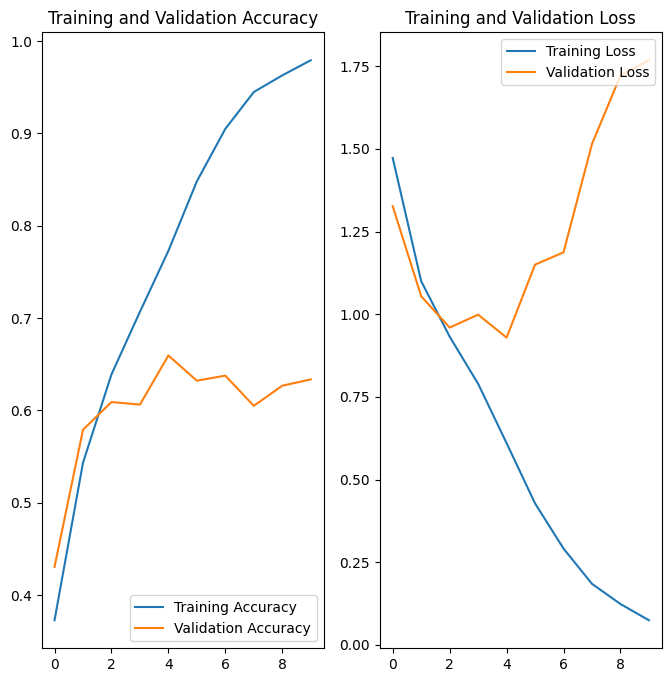The plots show that training accuracy and validation accuracy are off by large margins, and the model has achieved only around 60% accuracy on the validation set.

The following tutorial sections show how to inspect what went wrong and try to increase the overall performance of the model.

## Overfitting

In the plots above, the training accuracy is increasing linearly over time, whereas validation accuracy stalls around 60% in the training process. Also, the difference in accuracy between training and validation accuracy is noticeable—a sign of overfitting.

When there are a small number of training examples, the model sometimes learns from noises or unwanted details from training examples—to an extent that it negatively impacts the performance of the model on new examples. This phenomenon is known as overfitting. It means that the model will have a difficult time generalizing on a new dataset.

There are multiple ways to fight overfitting in the training process. In this tutorial, you'll use data augmentation and add dropout to your model.

## Data augmentation

Overfitting generally occurs when there are a small number of training examples. Data augmentation takes the approach of generating additional training data from your existing examples by augmenting them using random transformations that yield believable-looking images. This helps expose the model to more aspects of the data and generalize better.

You will implement data augmentation using the following Keras preprocessing layers: `tf.keras.layers.RandomFlip`, `tf.keras.layers.RandomRotation`, and `tf.keras.layers.RandomZoom`. These can be included inside your model like other layers, and run on the GPU.

``````data_augmentation = keras.Sequential(
[
layers.RandomFlip("horizontal",
input_shape=(img_height,
img_width,
3)),
layers.RandomRotation(0.1),
layers.RandomZoom(0.1),
]
)
``````

Visualize a few augmented examples by applying data augmentation to the same image several times:

``````plt.figure(figsize=(10, 10))
for images, _ in train_ds.take(1):
for i in range(9):
augmented_images = data_augmentation(images)
ax = plt.subplot(3, 3, i + 1)
plt.imshow(augmented_images.numpy().astype("uint8"))
plt.axis("off")
``````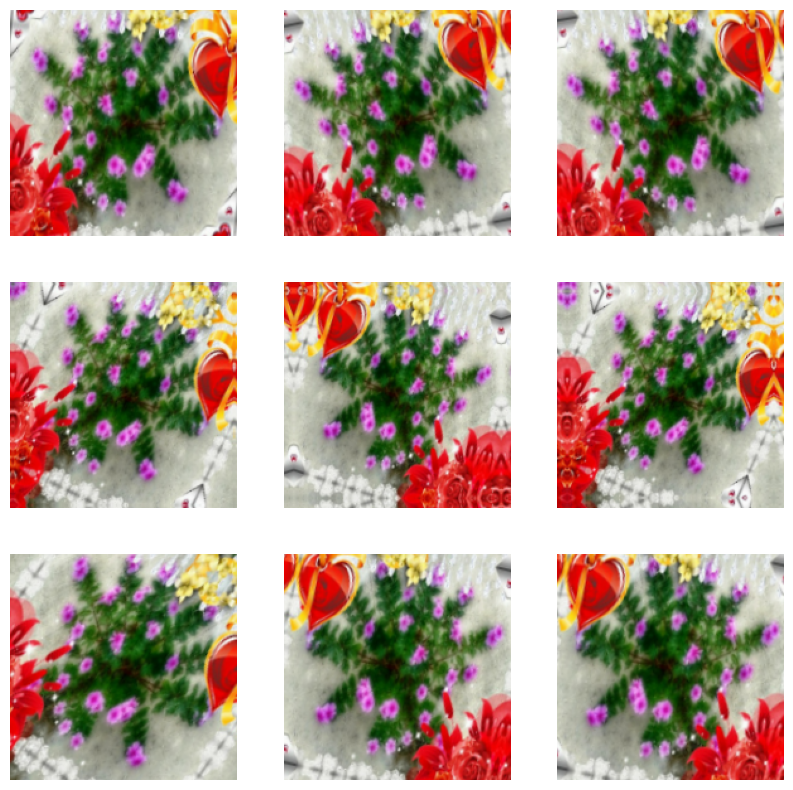You will add data augmentation to your model before training in the next step.

## Dropout

Another technique to reduce overfitting is to introduce dropout regularization to the network.

When you apply dropout to a layer, it randomly drops out (by setting the activation to zero) a number of output units from the layer during the training process. Dropout takes a fractional number as its input value, in the form such as 0.1, 0.2, 0.4, etc. This means dropping out 10%, 20% or 40% of the output units randomly from the applied layer.

Create a new neural network with `tf.keras.layers.Dropout` before training it using the augmented images:

``````model = Sequential([
data_augmentation,
layers.Rescaling(1./255),
layers.MaxPooling2D(),
layers.MaxPooling2D(),
layers.MaxPooling2D(),
layers.Dropout(0.2),
layers.Flatten(),
layers.Dense(128, activation='relu'),
layers.Dense(num_classes, name="outputs")
])
``````

## Compile and train the model

``````model.compile(optimizer='adam',
loss=tf.keras.losses.SparseCategoricalCrossentropy(from_logits=True),
metrics=['accuracy'])
``````
``````model.summary()
``````
```Model: "sequential_2"
_________________________________________________________________
Layer (type)                Output Shape              Param #
=================================================================
sequential_1 (Sequential)   (None, 180, 180, 3)       0

rescaling_2 (Rescaling)     (None, 180, 180, 3)       0

conv2d_3 (Conv2D)           (None, 180, 180, 16)      448

max_pooling2d_3 (MaxPoolin  (None, 90, 90, 16)        0
g2D)

conv2d_4 (Conv2D)           (None, 90, 90, 32)        4640

max_pooling2d_4 (MaxPoolin  (None, 45, 45, 32)        0
g2D)

conv2d_5 (Conv2D)           (None, 45, 45, 64)        18496

max_pooling2d_5 (MaxPoolin  (None, 22, 22, 64)        0
g2D)

dropout (Dropout)           (None, 22, 22, 64)        0

flatten_1 (Flatten)         (None, 30976)             0

dense_2 (Dense)             (None, 128)               3965056

outputs (Dense)             (None, 5)                 645

=================================================================
Total params: 3989285 (15.22 MB)
Trainable params: 3989285 (15.22 MB)
Non-trainable params: 0 (0.00 Byte)
_________________________________________________________________
```
``````epochs = 15
history = model.fit(
train_ds,
validation_data=val_ds,
epochs=epochs
)
``````
```Epoch 1/15
2023-10-27 06:07:14.991138: E tensorflow/core/grappler/optimizers/meta_optimizer.cc:961] layout failed: INVALID_ARGUMENT: Size of values 0 does not match size of permutation 4 @ fanin shape insequential_2/dropout/dropout/SelectV2-2-TransposeNHWCToNCHW-LayoutOptimizer
92/92 [==============================] - 5s 31ms/step - loss: 1.3151 - accuracy: 0.4441 - val_loss: 1.2732 - val_accuracy: 0.4837
Epoch 2/15
92/92 [==============================] - 3s 28ms/step - loss: 1.0668 - accuracy: 0.5725 - val_loss: 1.0116 - val_accuracy: 0.6076
Epoch 3/15
92/92 [==============================] - 3s 28ms/step - loss: 0.9600 - accuracy: 0.6287 - val_loss: 0.9160 - val_accuracy: 0.6403
Epoch 4/15
92/92 [==============================] - 3s 28ms/step - loss: 0.8789 - accuracy: 0.6570 - val_loss: 0.8687 - val_accuracy: 0.6621
Epoch 5/15
92/92 [==============================] - 3s 28ms/step - loss: 0.8106 - accuracy: 0.6935 - val_loss: 0.8116 - val_accuracy: 0.6921
Epoch 6/15
92/92 [==============================] - 3s 28ms/step - loss: 0.7825 - accuracy: 0.7033 - val_loss: 0.7915 - val_accuracy: 0.6798
Epoch 7/15
92/92 [==============================] - 3s 28ms/step - loss: 0.7277 - accuracy: 0.7224 - val_loss: 0.7513 - val_accuracy: 0.7084
Epoch 8/15
92/92 [==============================] - 3s 28ms/step - loss: 0.6826 - accuracy: 0.7408 - val_loss: 0.7406 - val_accuracy: 0.7016
Epoch 9/15
92/92 [==============================] - 3s 28ms/step - loss: 0.6711 - accuracy: 0.7371 - val_loss: 0.7415 - val_accuracy: 0.7084
Epoch 10/15
92/92 [==============================] - 3s 28ms/step - loss: 0.6370 - accuracy: 0.7582 - val_loss: 0.7297 - val_accuracy: 0.7193
Epoch 11/15
92/92 [==============================] - 3s 28ms/step - loss: 0.6310 - accuracy: 0.7537 - val_loss: 0.7839 - val_accuracy: 0.7003
Epoch 12/15
92/92 [==============================] - 3s 28ms/step - loss: 0.5819 - accuracy: 0.7769 - val_loss: 0.7163 - val_accuracy: 0.7139
Epoch 13/15
92/92 [==============================] - 3s 28ms/step - loss: 0.5743 - accuracy: 0.7810 - val_loss: 0.7068 - val_accuracy: 0.7248
Epoch 14/15
92/92 [==============================] - 3s 28ms/step - loss: 0.5292 - accuracy: 0.8065 - val_loss: 0.7622 - val_accuracy: 0.7330
Epoch 15/15
92/92 [==============================] - 3s 28ms/step - loss: 0.5136 - accuracy: 0.8072 - val_loss: 0.7274 - val_accuracy: 0.7343
```

## Visualize training results

After applying data augmentation and `tf.keras.layers.Dropout`, there is less overfitting than before, and training and validation accuracy are closer aligned:

``````acc = history.history['accuracy']
val_acc = history.history['val_accuracy']

loss = history.history['loss']
val_loss = history.history['val_loss']

epochs_range = range(epochs)

plt.figure(figsize=(8, 8))
plt.subplot(1, 2, 1)
plt.plot(epochs_range, acc, label='Training Accuracy')
plt.plot(epochs_range, val_acc, label='Validation Accuracy')
plt.legend(loc='lower right')
plt.title('Training and Validation Accuracy')

plt.subplot(1, 2, 2)
plt.plot(epochs_range, loss, label='Training Loss')
plt.plot(epochs_range, val_loss, label='Validation Loss')
plt.legend(loc='upper right')
plt.title('Training and Validation Loss')
plt.show()
``````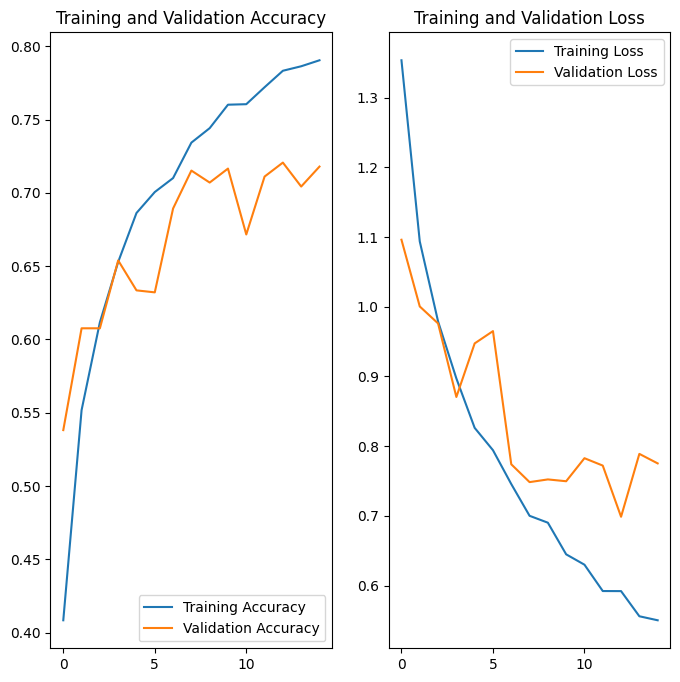## Predict on new data

Use your model to classify an image that wasn't included in the training or validation sets.

``````sunflower_url = "https://storage.googleapis.com/download.tensorflow.org/example_images/592px-Red_sunflower.jpg"
sunflower_path = tf.keras.utils.get_file('Red_sunflower', origin=sunflower_url)

sunflower_path, target_size=(img_height, img_width)
)
img_array = tf.keras.utils.img_to_array(img)
img_array = tf.expand_dims(img_array, 0) # Create a batch

predictions = model.predict(img_array)
score = tf.nn.softmax(predictions)

print(
"This image most likely belongs to {} with a {:.2f} percent confidence."
.format(class_names[np.argmax(score)], 100 * np.max(score))
)
``````
```Downloading data from https://storage.googleapis.com/download.tensorflow.org/example_images/592px-Red_sunflower.jpg
117948/117948 [==============================] - 0s 0us/step
1/1 [==============================] - 0s 252ms/step
This image most likely belongs to sunflowers with a 99.89 percent confidence.
```

## Use TensorFlow Lite

TensorFlow Lite is a set of tools that enables on-device machine learning by helping developers run their models on mobile, embedded, and edge devices.

### Convert the Keras Sequential model to a TensorFlow Lite model

To use the trained model with on-device applications, first convert it to a smaller and more efficient model format called a TensorFlow Lite model.

In this example, take the trained Keras Sequential model and use `tf.lite.TFLiteConverter.from_keras_model` to generate a TensorFlow Lite model:

``````# Convert the model.
converter = tf.lite.TFLiteConverter.from_keras_model(model)
tflite_model = converter.convert()

# Save the model.
with open('model.tflite', 'wb') as f:
f.write(tflite_model)
``````
```INFO:tensorflow:Assets written to: /tmpfs/tmp/tmplopz6g0i/assets
INFO:tensorflow:Assets written to: /tmpfs/tmp/tmplopz6g0i/assets
2023-10-27 06:07:58.906962: W tensorflow/compiler/mlir/lite/python/tf_tfl_flatbuffer_helpers.cc:378] Ignored output_format.
2023-10-27 06:07:58.906999: W tensorflow/compiler/mlir/lite/python/tf_tfl_flatbuffer_helpers.cc:381] Ignored drop_control_dependency.
Summary on the non-converted ops:
---------------------------------

* Accepted dialects: tfl, builtin, func
* Non-Converted Ops: 11, Total Ops 23, % non-converted = 47.83 %
* 11 ARITH ops

- arith.constant:   11 occurrences  (f32: 10, i32: 1)

(f32: 3)
(f32: 2)
(f32: 3)
(f32: 1)
```

The TensorFlow Lite model you saved in the previous step can contain several function signatures. The Keras model converter API uses the default signature automatically. Learn more about TensorFlow Lite signatures.

### Run the TensorFlow Lite model

You can access the TensorFlow Lite saved model signatures in Python via the `tf.lite.Interpreter` class.

Load the model with the `Interpreter`:

``````TF_MODEL_FILE_PATH = 'model.tflite' # The default path to the saved TensorFlow Lite model

interpreter = tf.lite.Interpreter(model_path=TF_MODEL_FILE_PATH)
``````

Print the signatures from the converted model to obtain the names of the inputs (and outputs):

``````interpreter.get_signature_list()
``````
```{'serving_default': {'inputs': ['sequential_1_input'], 'outputs': ['outputs']} }
```

In this example, you have one default signature called `serving_default`. In addition, the name of the `'inputs'` is `'sequential_1_input'`, while the `'outputs'` are called `'outputs'`. You can look up these first and last Keras layer names when running `Model.summary`, as demonstrated earlier in this tutorial.

Now you can test the loaded TensorFlow Model by performing inference on a sample image with `tf.lite.Interpreter.get_signature_runner` by passing the signature name as follows:

``````classify_lite = interpreter.get_signature_runner('serving_default')
classify_lite
``````
```<tensorflow.lite.python.interpreter.SignatureRunner at 0x7f93b01a1040>
```

Similar to what you did earlier in the tutorial, you can use the TensorFlow Lite model to classify images that weren't included in the training or validation sets.

You have already tensorized that image and saved it as `img_array`. Now, pass it to the first argument (the name of the `'inputs'`) of the loaded TensorFlow Lite model (`predictions_lite`), compute softmax activations, and then print the prediction for the class with the highest computed probability.

``````predictions_lite = classify_lite(sequential_1_input=img_array)['outputs']
score_lite = tf.nn.softmax(predictions_lite)
``````
``````print(
"This image most likely belongs to {} with a {:.2f} percent confidence."
.format(class_names[np.argmax(score_lite)], 100 * np.max(score_lite))
)
``````
```This image most likely belongs to sunflowers with a 99.89 percent confidence.
```

The prediction generated by the lite model should be almost identical to the predictions generated by the original model:

``````print(np.max(np.abs(predictions - predictions_lite)))
``````
```3.8146973e-06
```

Of the five classes—`'daisy'`, `'dandelion'`, `'roses'`, `'sunflowers'`, and `'tulips'`—the model should predict the image belongs to sunflowers, which is the same result as before the TensorFlow Lite conversion.

## Next steps

This tutorial showed how to train a model for image classification, test it, convert it to the TensorFlow Lite format for on-device applications (such as an image classification app), and perform inference with the TensorFlow Lite model with the Python API.